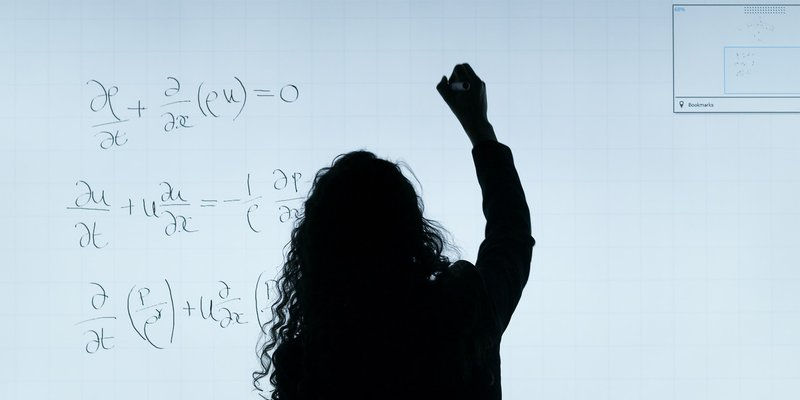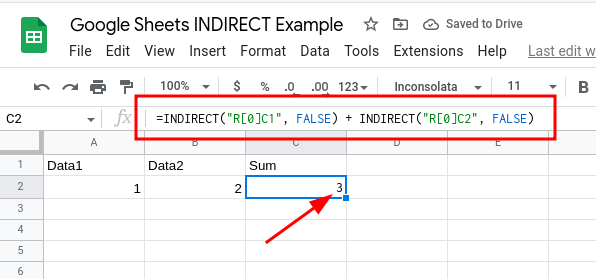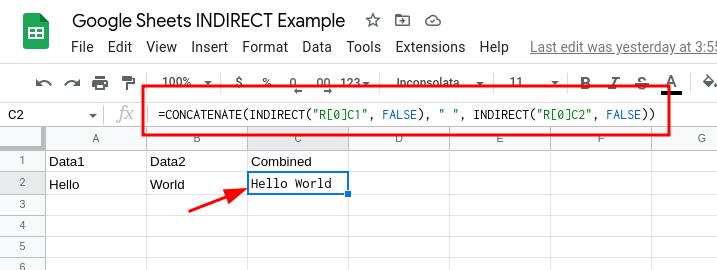## How to Reference the Current Row in Google Sheets FormulasGoogle Sheets has an INDIRECT function that lets users use relative row references in formulas.

For example, instead of using a formula like `=A2+B2`, you can replace the row numbers with a variable referencing the current row.  This way, you don't have to worry about whether the references for the current row are correct if your formula only deals with data in the current row.

This is particularly useful if you're pushing the formula to Google Sheets from an external app.

In the case of our app EZ Exporter, you can push Google Sheets formulas as cell values directly to the spreadsheet, but our app won't know which row number in the spreadsheet the data is pushed to when using the "append" option.  Our app will simply tell the Google Sheets API to append the data after the last row that contains data.

To reference the current row in the formula with the help of the INDIRECT function, we can use an R1C1 notation inside the function like this:

`=INDIRECT("RC1", FALSE) + INDIRECT("RC2", FALSE)`

This will add the values of columns 1 and 2 from the current row:

• "R" here stands for "Row" and "C" is for "Column."
• When the number is inside square brackets, this is the relative position. In this case, we're telling the formula to use the current row (0 rows below).  If you use "R", this means 1 row below the current row. A value of "R[-1]" would be 1 row above.
• If you don't use square brackets, the formula will use the absolute position. In the above example with "C1" and "C2", you're telling the function to always refer to column 1 and column 2 (i.e. columns A and B).
• The FALSE value in the second parameter specifies whether we're using A1 notation.  Since we want to use R1C1 notation, we set this to FALSE.As you can see above, we simply wrap the row and column references in the INDIRECT function.

Here's another example where we combine/concatenate the values from the first and second columns:

`=CONCATENATE(INDIRECT("RC1", FALSE), " ", INDIRECT("RC2", FALSE))`As you can see here, instead of `=CONCATENATE(A2, " ", B2)`, we replace A2 and B2 with the INDIRECT functions containing the relative row reference and absolute column references.

Related Posts: